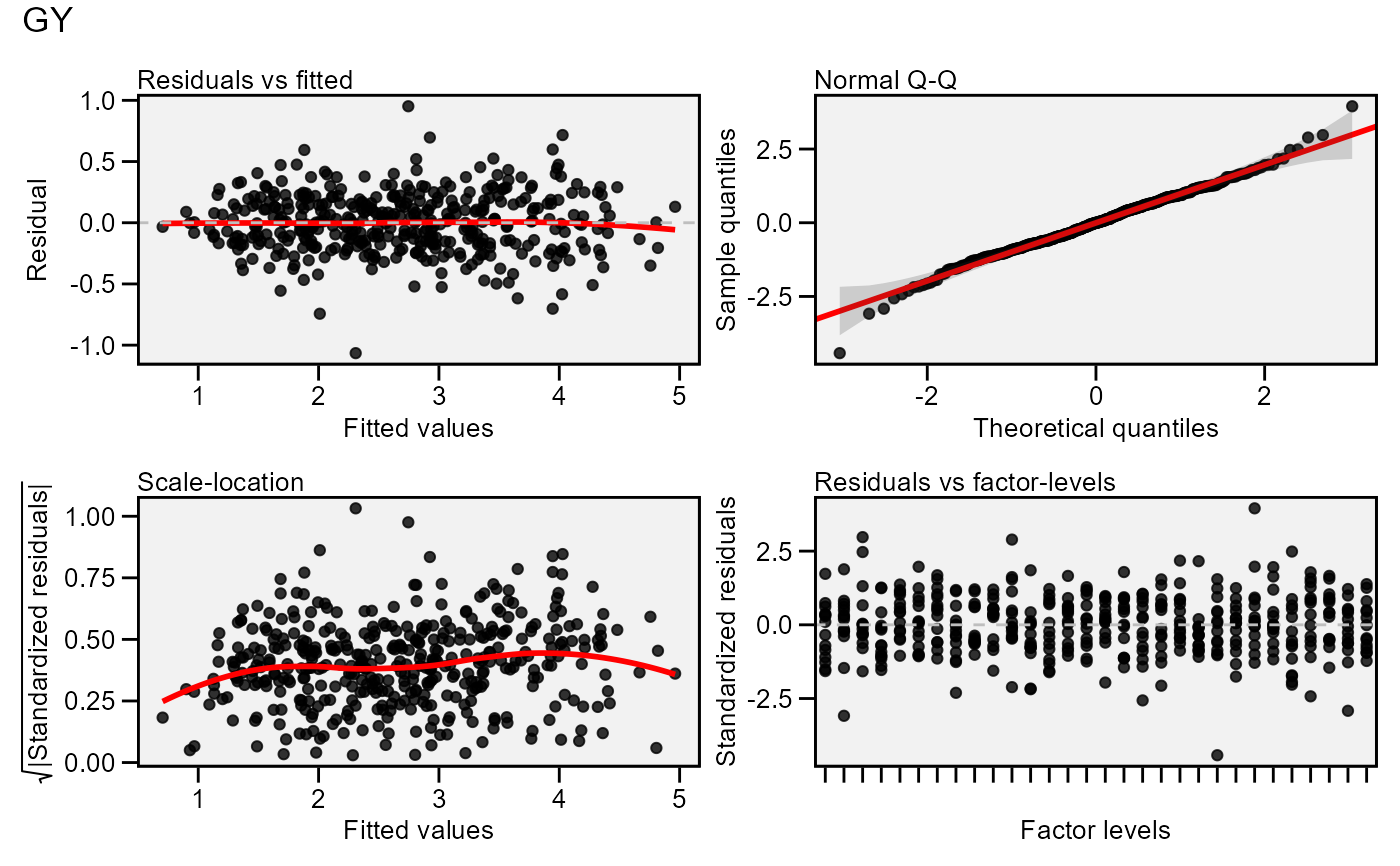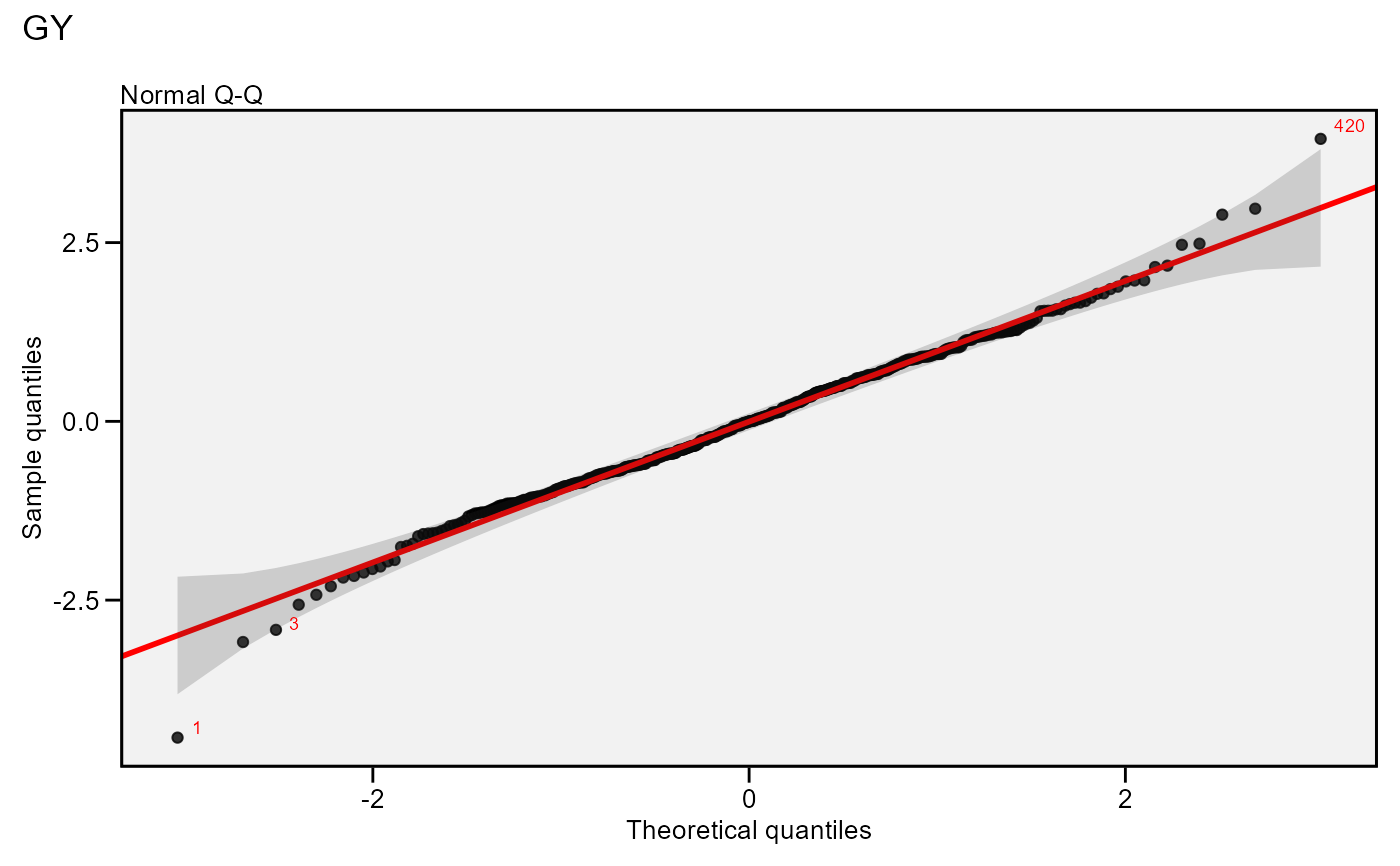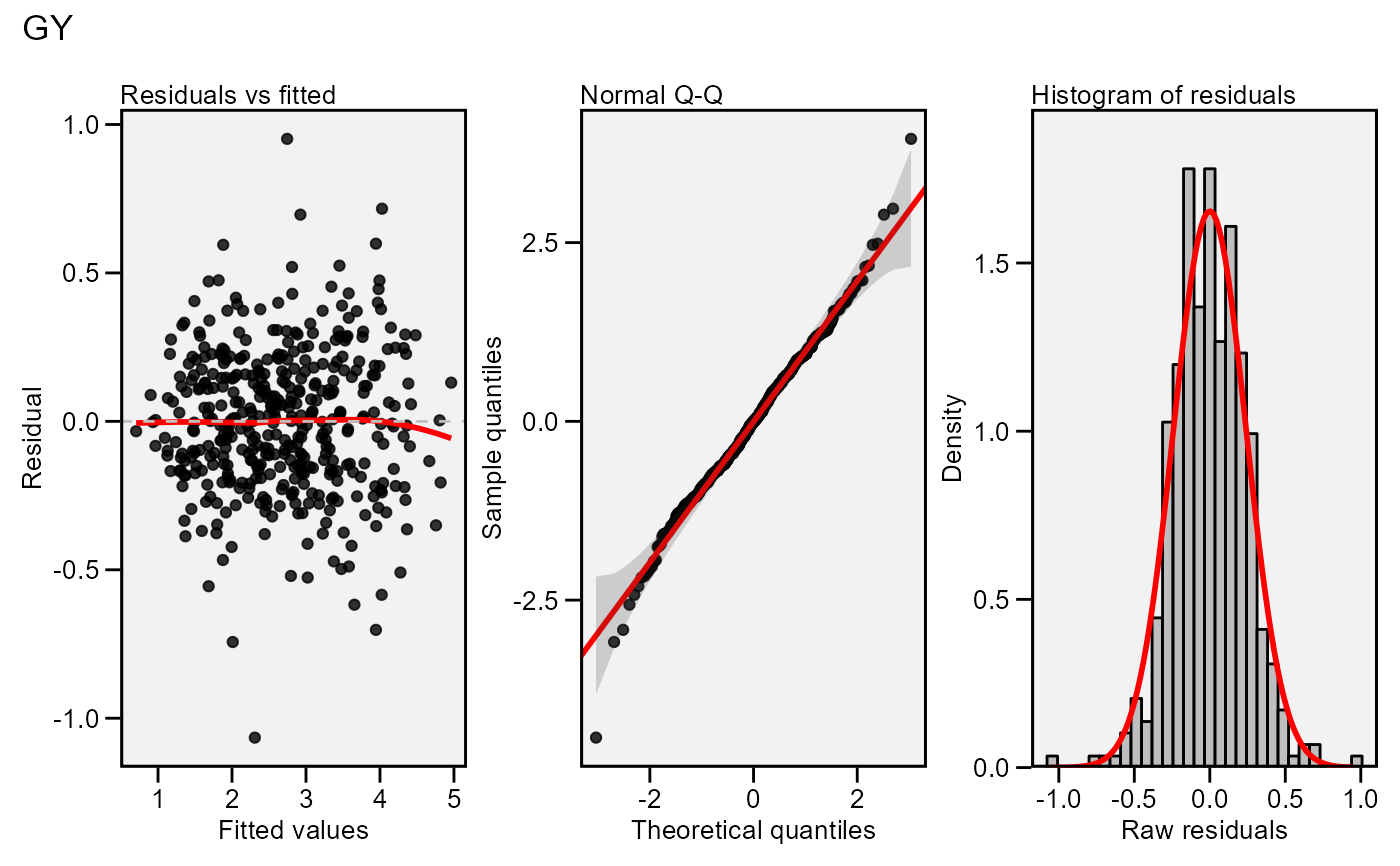Residual plots for a output model of class performs_ammi, waas, anova_ind, and anova_joint. Seven types of plots are produced: (1) Residuals vs fitted, (2) normal Q-Q plot for the residuals, (3) scale-location plot (standardized residuals vs Fitted Values), (4) standardized residuals vs Factor-levels, (5) Histogram of raw residuals and (6) standardized residuals vs observation order, and (7) 1:1 line plot

## Usage

residual_plots(
x,
var = 1,
conf = 0.95,
labels = FALSE,
plot_theme = theme_metan(),
band.alpha = 0.2,
point.alpha = 0.8,
fill.hist = "gray",
col.hist = "black",
col.point = "black",
col.line = "red",
col.lab.out = "red",
size.lab.out = 2.5,
size.tex.lab = 10,
size.shape = 1.5,
bins = 30,
which = c(1:4),
ncol = NULL,
nrow = NULL,
...
)

## Arguments

x

An object of class performs_ammi, waas, anova_joint, or gafem

var

The variable to plot. Defaults to var = 1 the first variable of x.

conf

Level of confidence interval to use in the Q-Q plot (0.95 by default).

labels

Logical argument. If TRUE labels the points outside confidence interval limits.

plot_theme

The graphical theme of the plot. Default is plot_theme = theme_metan(). For more details, see ggplot2::theme().

band.alpha, point.alpha

The transparency of confidence band in the Q-Q plot and the points, respectively. Must be a number between 0 (opaque) and 1 (full transparency).

fill.hist

The color to fill the histogram. Default is 'gray'.

col.hist

The color of the border of the the histogram. Default is 'black'.

col.point

The color of the points in the graphic. Default is 'black'.

col.line

The color of the lines in the graphic. Default is 'red'.

col.lab.out

The color of the labels for the 'outlying' points.

size.lab.out

The size of the labels for the 'outlying' points.

size.tex.lab

The size of the text in axis text and labels.

size.shape

The size of the shape in the plots.

bins

The number of bins to use in the histogram. Default is 30.

which

Which graphics should be plotted. Default is which = c(1:4) that means that the first four graphics will be plotted.

ncol, nrow

The number of columns and rows of the plot pannel. Defaults to NULL

...

Additional arguments passed on to the function patchwork::wrap_plots().

## Author

Tiago Olivoto tiagoolivoto@gmail.com

## Examples

# \donttest{
library(metan)
model <- performs_ammi(data_ge, ENV, GEN, REP, GY)
#> variable GY
#> ---------------------------------------------------------------------------
#> AMMI analysis table
#> ---------------------------------------------------------------------------
#>     Source  Df  Sum Sq Mean Sq F value   Pr(>F) Proportion Accumulated
#>        ENV  13 279.574 21.5057   62.33 0.00e+00         NA          NA
#>   REP(ENV)  28   9.662  0.3451    3.57 3.59e-08         NA          NA
#>        GEN   9  12.995  1.4439   14.93 2.19e-19         NA          NA
#>    GEN:ENV 117  31.220  0.2668    2.76 1.01e-11         NA          NA
#>        PC1  21  10.749  0.5119    5.29 0.00e+00       34.4        34.4
#>        PC2  19   9.924  0.5223    5.40 0.00e+00       31.8        66.2
#>        PC3  17   4.039  0.2376    2.46 1.40e-03       12.9        79.2
#>        PC4  15   3.074  0.2049    2.12 9.60e-03        9.8        89.0
#>        PC5  13   1.446  0.1113    1.15 3.18e-01        4.6        93.6
#>        PC6  11   0.932  0.0848    0.88 5.61e-01        3.0        96.6
#>        PC7   9   0.567  0.0630    0.65 7.53e-01        1.8        98.4
#>        PC8   7   0.362  0.0518    0.54 8.04e-01        1.2        99.6
#>        PC9   5   0.126  0.0252    0.26 9.34e-01        0.4       100.0
#>  Residuals 252  24.367  0.0967      NA       NA         NA          NA
#>      Total 536 389.036  0.7258      NA       NA         NA          NA
#> ---------------------------------------------------------------------------
#>
#> All variables with significant (p < 0.05) genotype-vs-environment interaction
#> Done!

# Default plot
plot(model)# Normal Q-Q plot
# Label possible outliers
plot(model,
which = 2,
labels = TRUE)# Residual vs fitted,
# Normal Q-Q plot
# Histogram of raw residuals
# All in one row
plot(model,
which = c(1, 2, 5),
nrow = 1)# }